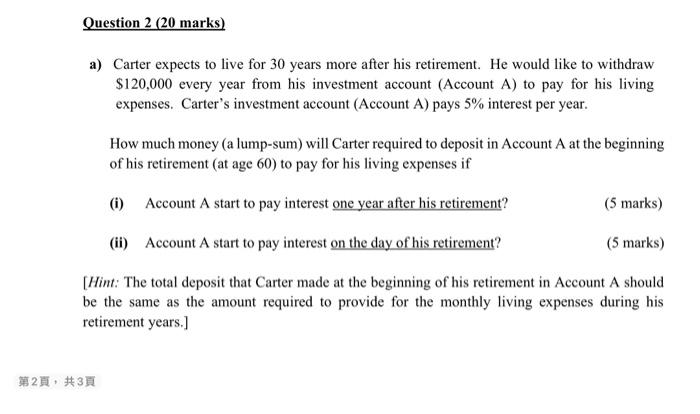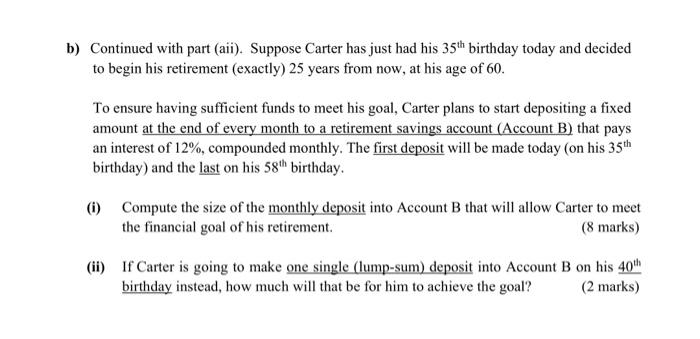# Question please help me to solve it  a) Carter expects to live for 30 years more after his retirement. He would like to withdraw $$\ 120,000$$ every year from his investment account (Account A) to pay for his living expenses. Carter's investment account (Account A) pays $$5 \%$$ interest per year. How much money (a lump-sum) will Carter required to deposit in Account $$\mathrm{A}$$ at the beginning of his retirement (at age 60 ) to pay for his living expenses if (i) Account A start to pay interest one year after his retirement? ( 5 marks) (ii) Account A start to pay interest on the day of his retirement? (5 marks) [Hint: The total deposit that Carter made at the beginning of his retirement in Account A should be the same as the amount required to provide for the monthly living expenses during his retirement years.] b) Continued with part (aii). Suppose Carter has just had his $$35^{\text {th }}$$ birthday today and decided to begin his retirement (exactly) 25 years from now, at his age of 60 . To ensure having sufficient funds to meet his goal, Carter plans to start depositing a fixed amount at the end of every month to a retirement savings account (Account B) that pays an interest of $$12 \%$$, compounded monthly. The first deposit will be made today (on his $$35^{\text {th }}$$ birthday) and the last on his $$58^{\text {th }}$$ birthday. (i) Compute the size of the monthly deposit into Account B that will allow Carter to meet the financial goal of his retirement. ( 8 marks) (ii) If Carter is going to make one single (lump-sum) deposit into Account B on his $$40^{\text {th }}$$ birthday instead, how much will that be for him to achieve the goal? ( 2 marks)Transcribed Image Text: a) Carter expects to live for 30 years more after his retirement. He would like to withdraw $$\ 120,000$$ every year from his investment account (Account A) to pay for his living expenses. Carter's investment account (Account A) pays $$5 \%$$ interest per year. How much money (a lump-sum) will Carter required to deposit in Account $$\mathrm{A}$$ at the beginning of his retirement (at age 60 ) to pay for his living expenses if (i) Account A start to pay interest one year after his retirement? ( 5 marks) (ii) Account A start to pay interest on the day of his retirement? (5 marks) [Hint: The total deposit that Carter made at the beginning of his retirement in Account A should be the same as the amount required to provide for the monthly living expenses during his retirement years.] b) Continued with part (aii). Suppose Carter has just had his $$35^{\text {th }}$$ birthday today and decided to begin his retirement (exactly) 25 years from now, at his age of 60 . To ensure having sufficient funds to meet his goal, Carter plans to start depositing a fixed amount at the end of every month to a retirement savings account (Account B) that pays an interest of $$12 \%$$, compounded monthly. The first deposit will be made today (on his $$35^{\text {th }}$$ birthday) and the last on his $$58^{\text {th }}$$ birthday. (i) Compute the size of the monthly deposit into Account B that will allow Carter to meet the financial goal of his retirement. ( 8 marks) (ii) If Carter is going to make one single (lump-sum) deposit into Account B on his $$40^{\text {th }}$$ birthday instead, how much will that be for him to achieve the goal? ( 2 marks)
Transcribed Image Text: a) Carter expects to live for 30 years more after his retirement. He would like to withdraw $$\ 120,000$$ every year from his investment account (Account A) to pay for his living expenses. Carter's investment account (Account A) pays $$5 \%$$ interest per year. How much money (a lump-sum) will Carter required to deposit in Account $$\mathrm{A}$$ at the beginning of his retirement (at age 60 ) to pay for his living expenses if (i) Account A start to pay interest one year after his retirement? ( 5 marks) (ii) Account A start to pay interest on the day of his retirement? (5 marks) [Hint: The total deposit that Carter made at the beginning of his retirement in Account A should be the same as the amount required to provide for the monthly living expenses during his retirement years.] b) Continued with part (aii). Suppose Carter has just had his $$35^{\text {th }}$$ birthday today and decided to begin his retirement (exactly) 25 years from now, at his age of 60 . To ensure having sufficient funds to meet his goal, Carter plans to start depositing a fixed amount at the end of every month to a retirement savings account (Account B) that pays an interest of $$12 \%$$, compounded monthly. The first deposit will be made today (on his $$35^{\text {th }}$$ birthday) and the last on his $$58^{\text {th }}$$ birthday. (i) Compute the size of the monthly deposit into Account B that will allow Carter to meet the financial goal of his retirement. ( 8 marks) (ii) If Carter is going to make one single (lump-sum) deposit into Account B on his $$40^{\text {th }}$$ birthday instead, how much will that be for him to achieve the goal? ( 2 marks)&#12304;General guidance&#12305;The answer provided below has been developed in a clear step by step manner.Step1/2a) (i) To calculate the lump-sum amount that Carter needs to deposit in Account A to withdraw $120,000 annually for 30 years starting one year after his retirement at 60, we can use the present value formula:PV = PMT x ((1 - (1 + r)^-n) / r)where PV is the present value or lump-sum amount, PMT is the annual payment, r is the interest rate, and n is the number of periods.In this case, PMT =$120,000, r = 5%, and n = 30.PV = $120,000 x ((1 - (1 + 0.05)^-30) / 0.05) =$1,746,717.55.Therefore, Carter will need to deposit approximately $1,746,718 in Account A to meet his retirement expenses.(ii) If Account A starts paying interest on the day of his retirement, we need to calculate the present value of an annuity due, which means that the payments are made at the beginning of each period instead of the end. The formula for the present value of an annuity due is:PV = PMT x ((1 - (1 + r)^-n) / r) x (1 + r)where PV is the present value, PMT is the payment, r is the interest rate, n is the number of periods.In this case, PMT =$120,000, r = 5%, and n = 30.PV = $120,000 x ((1 - (1 + 0.05)^-30) / 0.05) =$1,746,717.55Therefore, Carter will need ... See the full answer